Study Materials

# NCERT Solutions for Class 6th Mathematics

Page 1 of 3

## Chapter 6. Integers

### Exercise 6.1

Exercise 6.1

Q1. Write opposites of the following :

(a) Increase in weight

(b) 30 km north

(c) 326 BC

(d) Loss of Rs 700

(e) 100 m above sea level

Solution: Q1.

(a) Increase in weight

(b) 30 km North

(c) 326 BC

(d) Loss of Rs 700

Answer : Profit of Rs 700.

(e) 100 m above sea level

Answer : 100 m below sea level.

Q2. Represent the following numbers as integers with appropriate signs.
(a) An aeroplane is flying at a height two thousand metre above the
ground.
(b) A submarine is moving at a depth, eight hundred metre below the sea level.
(c) A deposit of rupees two hundred.
(d) Withdrawal of rupees seven hundred.

Solution Q2.

(a) An aeroplane is flying at a height two thousand metre above the
ground.

(b) A submarine is moving at a depth, eight hundred metre below the sea level.

(c) A deposit of rupees two hundred.

(d) Withdrawal of rupees seven hundred.

Q3. Represent the following numbers on a number line :

(a) + 5

(b) – 10

(c) + 8

(d) – 1

(e) – 6

Solution Q3:

(a) + 5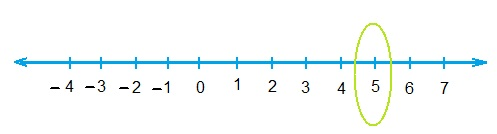(b) – 10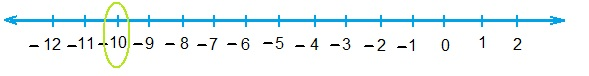(c) + 8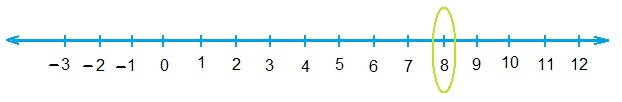(d) – 1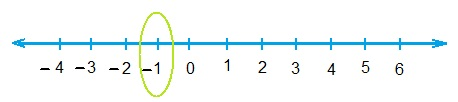(e) – 6Q4. Adjacent figure is a vertical number line, representing integers. Observe it and locate the following points :
(a) If point D is + 8, then which point is – 8?(b) Is point G a negative integer or a positive integer?
(c) Write integers for points B and E.
(d) Which point marked on this number line has the least value?
(e) Arrange all the points in decreasing order of value.

Solution Q4.

(a) If point D is + 8, then which point is – 8?

(b) Is point G a negative integer or a positive integer?

(c) Write integers for points B and E.

(i) For point B : + 4

(ii) For point E : - 10

(d) Which point marked on this number line has the least value?

Answer : Point E has least value.

(e) Arrange all the points in decreasing order of value.

Answer : D, C, B, A, O, H, G, F, E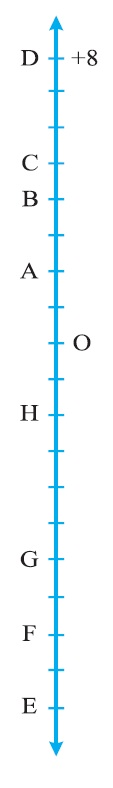Q5. Following is the list of temperatures of five places in India on a particular day of the year.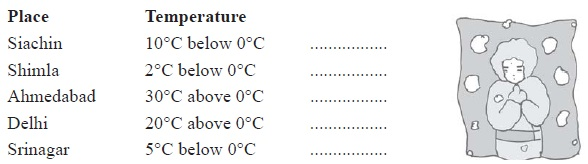(a) Write the temperatures of these places in the form of integers in the blank column.
(b) Following is the number line representing the temperature in degree Celsius.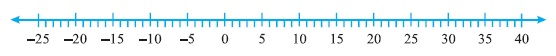Plot the name of the city against its temperature.
(c) Which is the coolest place?
(d) Write the names of the places where temperatures are above 10°C.

Solution Q5:

(a) Write the temperatures of these places in the form of integers in the blank column.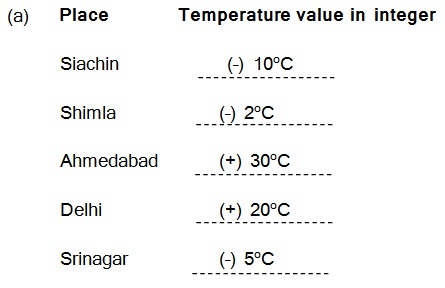(b) Following is the number line representing the temperature in degree Celsius.

Plot the name of the city against its temperature.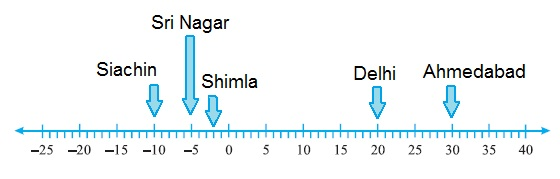(c) Which is the coolest place?

Answer : The coolest place is Siachin

(d) Write the names of the places where temperatures are above 10°C.

Q6. In each of the following pairs, which number is to the right of the other on the number line?

(a) 2, 9

(b) – 3, – 8

(c) 0, – 1

(d) – 11, 10

(e) – 6, 6

(f) 1, – 100

Solution Q6:

(a) 9 is right to 2

(b) –3 is right to –8

(c) 0 is right to –1

(d) 10 is right to –11

(e) 6 is right to –6

(f) 1 is right to –100

Q7. Write all the integers between the given pairs (write them in the increasing order.)
(a) 0 and – 7

(b) – 4 and 4

(c) – 8 and – 15

(d) – 30 and – 23

Solution Q7:

(a) 0 and – 7

Answer : –6, –5, –4, –3, –2, –1

(b) – 4 and 4

Answer :  –3, –2, –1, 0, 1, 2, 3

(c) – 8 and – 15

Answer :  –14, –13, –12, –11, –10, –9

(d) – 30 and – 23

Answer : –29, –28, –27, –26, –25, –24

Q8. (a) Write four negative integers greater than – 20.

Solution : –19, –18, –17, –16

(b) Write four negative integers less than – 10.

Solution :  –11, –12, –13, –14

Q9. For the following statements, write True (T) or False (F). If the statement is false, correct the statement.

(a) – 8 is to the right of – 10 on a number line.

Solution : True (T)

(b) – 100 is to the right of – 50 on a number line.

Solution : False (F)

(c) Smallest negative integer is – 1.

Solution: False (F)

(d) – 26 is greater than – 25.

Solution: False (F)

Q10. Draw a number line and answer the following :

(a) Which number will we reach if we move 4 numbers to the right of – 2.

Solution :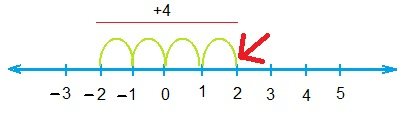(b) Which number will we reach if we move 5 numbers to the left of 1.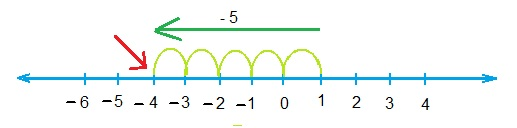(c) If we are at – 8 on the number line, in which direction should we move to reach – 13?

Solution: We should move to left side.

(d) If we are at – 6 on the number line, in which direction should we move to reach – 1?

Solution: We should move to right side.

Page 1 of 3

Chapter Contents: